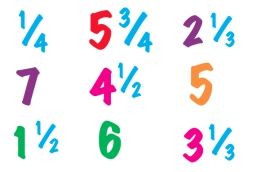# The sum 34

The sum of two fractions is 5/6. One of the fractions is 1/2. What is the other fraction?

b =  1/3

### Step-by-step explanation:Did you find an error or inaccuracy? Feel free to write us. Thank you!

Tips for related online calculators
Need help calculating sum, simplifying, or multiplying fractions? Try our fraction calculator.